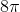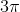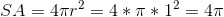## Example Questions

### Example Question #1 : How To Find The Surface Area Of A Sphere

What is the surface area of a composite figure of a cone and a sphere, both with a radius of 5 cm, if the height of the cone is 12 cm? Consider an ice cream cone as an example of the composite figure, where half of the sphere is above the edge of the cone.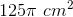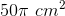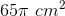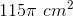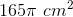Explanation:

Calculate the slant height height of the cone using the Pythagorean Theorem. The height will be the height of the cone, the base will be the radius, and the hypotenuse will be the slant height.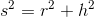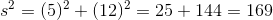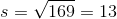The surface area of the cone (excluding the base) is given by the formula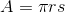. Plug in our values to solve.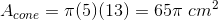The surface area of a sphere is given by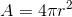but we only need half of the sphere, so the area of a hemisphere is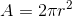.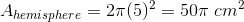So the total surface area of the composite figure is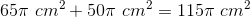.

### Example Question #2 : How To Find The Surface Area Of A Sphere

The volume of a sphere is found using the formula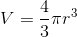.

The surface area of a sphere is found using the formula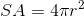.

Suppose a sphere has a surface area of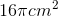. What is its volume?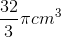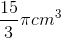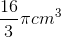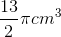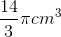Explanation:

The first step is to use the surface area formula to find the radius of the sphere.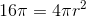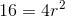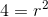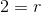The next step is to plug the value of the radius into the volume formula.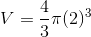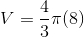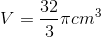### Example Question #3 : How To Find The Surface Area Of A Sphere

What is the surface area of a sphere with a diameter, in centimeters,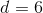The surface area (SA) of a sphere is calculated using the formula?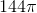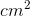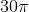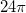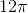Explanation:

If, then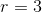. Plug the radius into the equation for surface area to get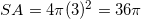.

### Example Question #4 : How To Find The Surface Area Of A Sphere

What is the surface area of a sphere that has a diameter of eight inches? Reduce any fractions in your answer and leave your answer in terms of.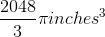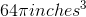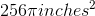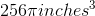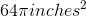Explanation:

To find the volume of a sphere area of a sphere plug the radius into the following formula given by :.

To find the radius given the diameter, divide the diameter by 2.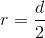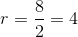Thus: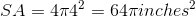### Example Question #5 : How To Find The Surface Area Of A Sphere

What is the surface area of a sphere, in inches, that has a surface area equal to its volume? Leave your answer in terms of.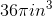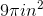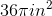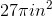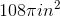Explanation:

To find the radius of a sphere that has a volume equal to it's surface area, begin by setting the volume and surface area formulas for a sphere equal to each other and solving for the radius: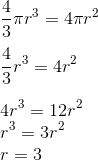Next we plug the answer for our radius into the formula for the surface area: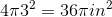, remember to check the units.

### Example Question #6 : How To Find The Surface Area Of A Sphere

Find the surface area of a sphere whose radius is.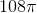Explanation:

To solve, simply remember the following formula: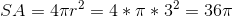### Example Question #7 : How To Find The Surface Area Of A Sphere

Find the surface area of a sphere whose radius is. Thus,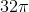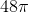Explanation:

To find surface area, simply use the formula. Thus,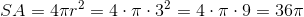### Example Question #8 : How To Find The Surface Area Of A Sphere

Find the surface area of a sphere whose side diameter is.